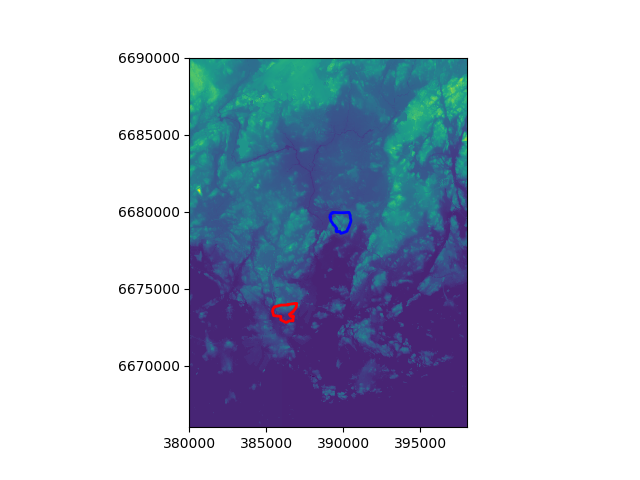# Zonal statistics¶

Quite often you have a situtation when you want to summarize raster datasets based on vector geometries. Rasterstats is a Python module that does exactly that, easily.

In : import rasterio

In : from rasterio.plot import show

In : from rasterstats import zonal_stats

In : import osmnx as ox

In : import geopandas as gpd

• Specify filepath, this is the mosaic raster file that was created earlier.
In : dem_fp = r"C:\HY-DATA\HENTENKA\KOODIT\Opetus\Automating-GIS-processes\Data\CSC_Lesson6\Helsinki_DEM_2x2m_Mosaic.tif"

• Read in the DEM data
In : dem = rasterio.open(dem_fp)

In : kallio_q = "Kallio, Helsinki, Finland"

In : pihlajamaki_q = "Pihlajamäki, Malmi, Helsinki, Finland"

• Retrieve ‘Kallio’ and ‘Pihlajamäki’ regions from OpenStreetMap
In : kallio = ox.gdf_from_place(kallio_q)

In : pihlajamaki = ox.gdf_from_place(pihlajamaki_q)

• Reproject the regions to same CRS as the DEM
In : kallio = kallio.to_crs(crs=dem.crs.data)

In : pihlajamaki = pihlajamaki.to_crs(crs=dem.crs.data)

• Plot the DEM and the regions on top of it
In : ax = show((dem, 1))

In : kallio.plot(ax=ax, facecolor='None', edgecolor='red', linewidth=2)
Out: <matplotlib.axes._subplots.AxesSubplot at 0x20c92beb278>

In : pihlajamaki.plot(ax=ax, facecolor='None', edgecolor='blue', linewidth=2)
Out: <matplotlib.axes._subplots.AxesSubplot at 0x20c92beb278>Which one is higher? Kallio or Pihlajamäki? We can use zonal statistics to find out!

• First we need to get the values of the dem as numpy array and the affine of the raster
In : array = dem.read(1)

In : affine = dem.affine

• Now we can calculate the zonal statistics by using the function zonal_stats.
In : zs_kallio = zonal_stats(kallio, array, affine=affine, stats=['min', 'max', 'mean', 'median', 'majority'])

In : zs_pihla = zonal_stats(pihlajamaki, array, affine=affine, stats=['min', 'max', 'mean', 'median', 'majority'])


Okey. So what do we have now?

In : print(zs_kallio)
[{'min': -2.1760001182556152, 'max': 37.388999938964844, 'mean': 12.96425898303966, 'median': 11.75100040435791, 'majority': 0.3490000069141388}]

In : print(zs_pihla)
[{'min': 8.73799991607666, 'max': 46.30400085449219, 'mean': 24.560033970865877, 'median': 24.17300033569336, 'majority': 10.41100025177002}]


Super! Now we can see that Pihlajamäki seems to be slightly higher compared to Kallio.